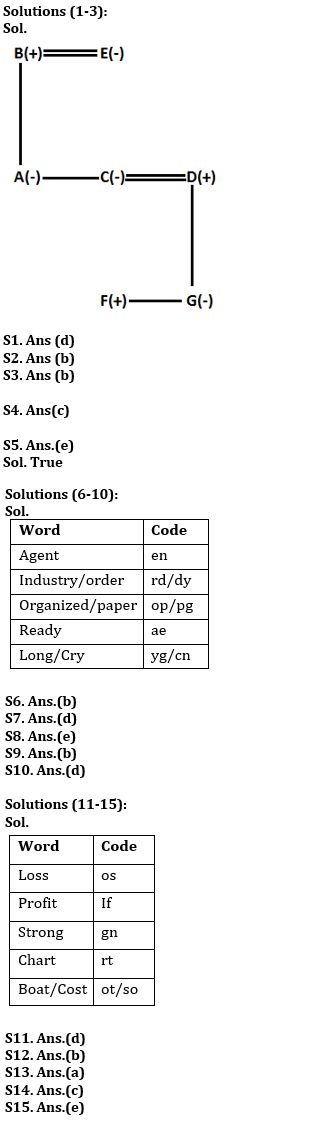Latest Banking jobs   »   Reasoning Ability Quiz For Bank Foundation...

# Reasoning Ability Quiz For Bank Foundation 2023 -03rd May

Directions (1-3): Study the following information and answer the given questions:
In a family of seven members, there is only two married couple. A is daughter of B who is husband of E. D is married to C who is sister of A. B has no son. E is grandmother of F. G is the only daughter of D. F is the son of C.

Q1. How A is related to D?
(a) Daughter
(b) Cousin
(c) Niece
(d) Sister-in-law
(e) Cannot be determined

Q2. How F is related to B?
(a) Brother
(b) Grandson
(c) Granddaughter
(d) Sister
(e) none of these

Q3. How many male members are there in the family?
(a) One
(b) Three
(c) Two
(d) Cannot be determined
(e) None of these

Q4. Pointing to a lady, Sumit says to his only son, “She is daughter in law of my mother’s daughter in law”. Sumit is the only Child of his parents. How is the lady related to Sumit?
(a) Sister
(b) Brother
(c) Daughter-in-law
(d) Wife
(e) Daughter

Q5. Which of the following is the third letter from the right end of the meaningful word formed from the 1st, 5th, 6th and 9th letters of the word “UNIVERSITY”. If more than one word is formed your answer will be Z.
(a) U
(b) E
(c) Z
(d) T
(e) R

Directions (6-10): Study the following information carefully and answer the questions given below:
In a certain code language:
‘Agent Industry Order’ is written as ‘rd en dy’,
‘Organized Agent Paper’ is written as ‘op pg en’,
‘Industry Ready Order’ is written as ‘dy rd ae’
‘Agent Long Cry’ is written as ‘en yg cn’.

Q6. What is the code for ‘Ready’ in the given code language?
(a) rd
(b) ae
(c) dy
(d) cn
(e) None of these

Q7. If ‘Organized’ is coded as ‘pg’. What is the code for ‘Bank Paper’ in the given code language?
(a) op ae
(b) yg pg
(c) yg op
(d) op kb
(e) None of these

Q8. What is the code for ‘Industry’ in the given code language?
(a) en
(b) pg
(c) rd
(d) dy
(e) Can’t be determined

Q9. What may be the code for ‘order Smart Cry’ in the given code language?
(a) rd mr ew
(b) dy yg mr
(c) yg cn mr
(d) cn yg rd
(e) None of these

Q10. What may be the code for ‘long’?
(a) op
(b) cn
(c) yg
(d) Either (b) or (c)
(e) None of these

Directions (11-15): Study the following information carefully and answer the questions given below:
In a certain code language:
‘Loss Profit Strong’ is written as ‘os if gn’,
‘Profit Chart Strong’ is written as ‘rt gn if’,
‘Boat Profit Cost’ is written as ‘if ot so’
‘Strong Chart Profit’ is written as ‘gn rt if’.

Q11. What is the code for ‘Chart’ in the given code language?
(a) gn
(b) if
(c) ot
(d) rt
(e) None of these

Q12. If ‘Boat’ is coded as ‘so’. What is the code for ‘article cost’ in the given code language?
(a) le if
(b) ot le
(c) so gn
(d) if rt
(e) None of these

Q13. What is the code for ‘Profit’ in the given code language?
(a) if
(b) gn
(c) ot
(d) rt
(e) Can’t be determined

Q14. What may be the code for ‘Strong’ in the given code language?
(a) if
(b) rt
(c) gn
(d) so
(e) None of these

Q15. What may be the code for ‘Loss Chart cost’?
(a) so gn ot
(b) if gn ot
(c) os gn ot
(d) so gn if
(e) None of these

Solutions## FAQs

### What is the selection process of the Bank Clerk?

The selection process of the Bank Clerk is Prelims & Mains.

#### Congratulations!Union Budget 2023-24: Free PDF